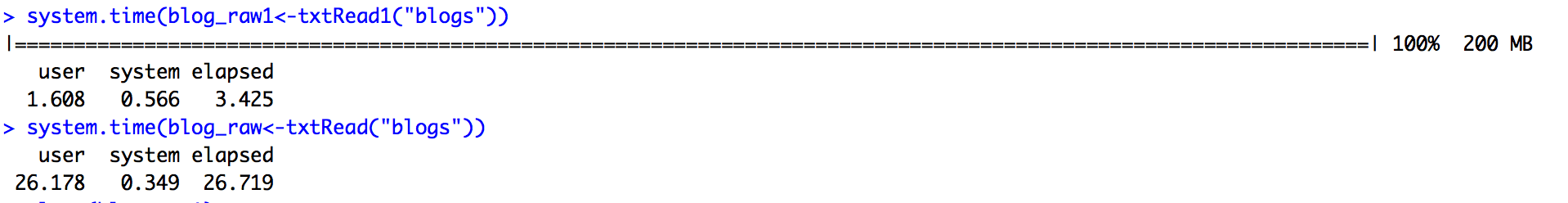# Coursera Data Science Specialization Capstone course learning journal 2 – Reading .txt file with R

Reading the large .txt files from the course project has been a long learning journey for me.

# Method 1: R base function: `readLines()`

I first started using the R base function readLines(). This would return a character vector, which the length() function can be used on to count the number of lines.

```txtRead1<-function(x){ path_name<-getwd() path<-paste(path_name,"/final/en_US/en_US.",x,".txt",sep="") txt_file<-readLines(path, encoding = "UTF-8") return(txt_file) }```

# Method 2: `readtext()` function

I then started reading about Quanteda and learned that readtext works well with `Quanteda`. So I installed the readtext package, and used it for reading the .txt files. The output file would be a 2-column one row data.frame by default. However, using the docvarsfrom, docvarnames, and dvsep, one can parse the file name, file path, and pass the meta information to the output data frame as additional columns. For example, the following information allowed me to add two additional columns of “language” and “type” by parsing the file names.

``` txtRead<-function(x){ path_name<-getwd() path<-paste(path_name,"/final/en_US/en_US.",x,".txt",sep="") txt_file<-readtext(path,docvarsfrom = "filenames", docvarnames = c("language", "type"), dvsep = "[.]", encoding = "UTF-8") return(txt_file) } ```

Using `length() `on the output from `readtext()` would result in a number “4” on the entire data. frame, or number “1” on the variable “text. ”I was then able to use `object.size() `to get the output file’s size, `sum(nchar())` to get the total number of characters, and `ntoken()` to get total number of words. However, readtext() would collapse all text lines together, and therefore I couldn’t use the `length()` function to count the number of lines anymore.

# Method 3: `readr()` function

I thought of going back to `readr()` and happily found that `readr()` seems to be much fast than `readLines()`. See below, txtRead1 is the function using readLines and txtRead uses `readr()`. Yet they both return a long character vector.However, using both `readr()` and `readLines()` still feel awkward, especially thinking of the following step of creating corpus.

After reading more about the philosophy of `Quanteda()`, about the definition of Corpus which is to preserve the original information as much as possible, I decided to give the line length method another try. Searching around a bit more, I found that this simple R base function “str_count” would do the trick:

So below is the full line about getting file size, line counts, word counts, and char counts:

```textStats <- data.frame('Type' = c("Blog","News","Twitter"), "File Size" = sapply(list(blog_raw, news_raw, twitter_raw), function(x){object.size(x\$text)}), 'Lines' = sapply(list(blog_raw, news_raw, twitter_raw), function(x){str_count(x\$text,"\\n")+1}), 'Total Words' = sapply(list(blog_raw, news_raw, twitter_raw),function(x){sum(ntoken(x\$text))}), 'Total Chars' = sapply(list(blog_raw, news_raw, twitter_raw), function(x){sum(nchar(x\$text))}) )```

Next journal would talk about creating a grouped bar chart using `Plotly()`.